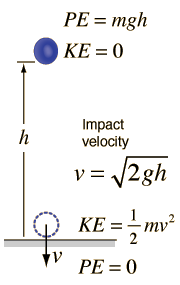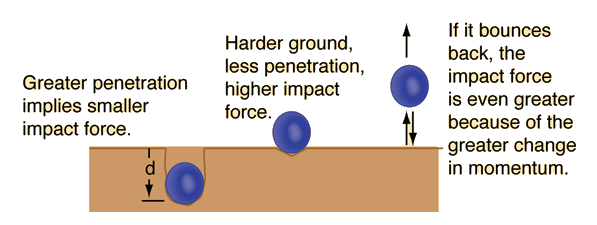# Impact Force from Falling Object

Even though the application of conservation of energy to a falling object allows us to predict its impact velocity and kinetic energy, we cannot predict its impact force without knowing how far it travels after impact.If an object of mass m=kg is dropped from height h = m, then the velocity just before impact is v = m/s. The kinetic energy just before impact is equal to its gravitational potential energy at the height from which it was dropped: K.E. = J. But this alone does not permit us to calculate the force of impact! If in addition, we know that the distance traveled after impact is d =m, then the impact force may be calculated using the work-energy principle to be Average impact force = F = N.Note that the above calculation of impact force is accurate only if the height h includes the stopping distance, since the process of penetration is further decreasing its gravitational potential energy.

The average impact force calculated here is the average over distance, which can be presumed to be proportional to, but not the same as, the average over time.

Index

 HyperPhysics***** Mechanics R Nave
Go Back